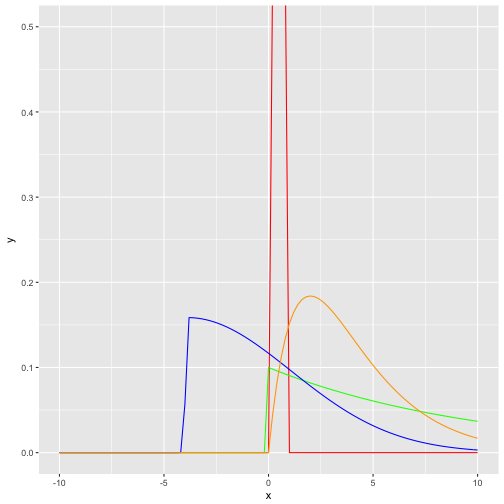# Plotting skewed distributions

## April 19, 2017

Reading time ~3 minutes

Let’s plot some skewed stuff, aehm, distributions!

Actually, the point I - initially - wanted to make is that in skewed distribution, don’t use means. Or at least, be very aware that (arithmetic) means can be grossly misleading. But for today, let’s focus on drawing skewed distributions.

Some packages:

library(tidyverse)
library(fGarch) # for snorm


Some skewed distribution include:

• “polluted” normal distributions, ie., mixtures of two normals
• Exponential distributions
• Gamma distributions
• Beta distributions

One way to visualize them is to draw their curve, ie., their functional (analytical) form:

data_frame(
x = seq(-10, 10, .05)
) %>%
ggplot +
aes(x) +
stat_function(fun = dbeta, args = list(shape1 = 4, shape2 = 4), color = "red") +
stat_function(fun = dexp, args = list(rate = .10), color = "green") +
stat_function(fun = dsnorm, args = list(mean = 0, sd = 3, xi = 7.5), color = "blue") +
stat_function(fun = dgamma, args = list(shape = 2, scale = 2), color = "orange") +
coord_cartesian(ylim = c(0,.5))Second, we could draw some random instances from the respective distribution; we will get then not “smooth” curves but more “realistic” or “zigzag” histogram (or density diagrams).

df <- data_frame(
skewed_normal = rsnorm(n = 1000, mean = 0, sd = 18, xi = 130),
exp_distrib = rexp(n = 1000, rate = .1),
gamma_distrib = rgamma(n = 1000, shape = 2, scale = 2),
beta_distrib = rbeta(n = 1000, shape1 = 4, shape2 = 2)

)

mypal <-

df %>%
gather(key = distribution, value = value) %>%
ggplot +
aes(x = value) +
geom_histogram(aes(fill = distribution)) +
facet_wrap(~distribution) +
scale_fill_manual(values = c("red", "green", "blue", "orange")) +
scale_color_manual(values = c("red", "green", "blue", "orange")) +
labs(title = "Histogram of random draws from different distributions",
subtitle = "test")


Note that the domain of the beta distribution is [0,1], that’s why a narrow red bar pops out as histogram (the other distribution spread out much more explicitly). See:

df %>%
gather(key = distribution, value = value) %>%
dplyr::filter(distribution == "beta_distrib") %>%
ggplot +
aes(x = value) +
geom_histogram(aes(fill = distribution)) +
#facet_wrap(~distribution) +
scale_fill_manual(values = c("red", "green", "blue", "orange")) +
scale_color_manual(values = c("red", "green", "blue", "orange")) +
labs(title = "Histogram of random draws from a Beta distribution")

## stat_bin() using bins = 30. Pick better value with binwidth.### This blog has moved

This blog has moved to Adios, Jekyll. Hello, Blogdown!… Continue reading

#### Wie gut schätzt eine Stichprobe die Grundgesamtheit?

Published on November 17, 2017

#### Some thoughts on tidyveal and environments in R

Published on November 16, 2017